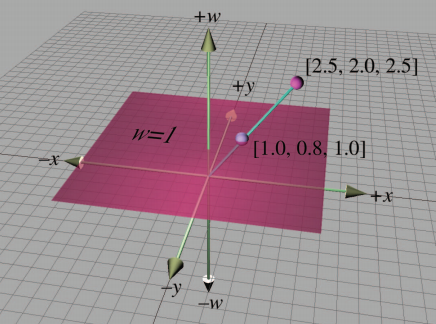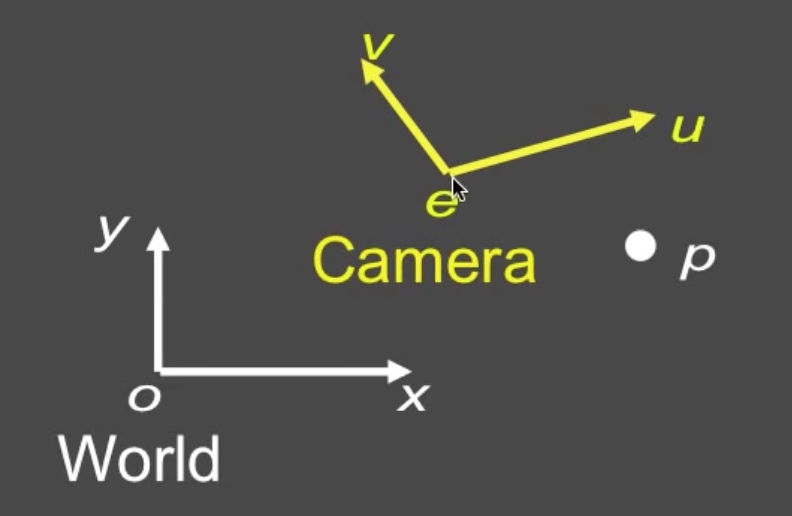2020年7月26日更新：

## 平移（Translation）

$\begin{bmatrix}x' \\ y' \\ z' \end{bmatrix}=\begin{bmatrix} & & \\ & ? & \\ & & \end{bmatrix}\begin{bmatrix}x \\ y \\ z \end{bmatrix}=\begin{bmatrix}x+5 \\ y \\ z \end{bmatrix}$

$\begin{bmatrix}x' \\ y' \\ z' \\ w' \end{bmatrix}=\begin{bmatrix} 1 & 0 & 0 & 5\\ 0 & 1 & 0 & 0\\ 0 & 0 & 1 & 0\\ 0 & 0 & 0 & 1 \end{bmatrix}\begin{bmatrix}x \\ y \\ z \\ 1 \end{bmatrix}=\begin{bmatrix}x+5 \\ y \\ z \\ 1 \end{bmatrix}$

## 齐次坐标

—— F.S. Hill, JR 《计算机图形学(OpenGL 版)》作者$\begin{bmatrix}x \\ y \\ z \\ w \end{bmatrix}=\begin{bmatrix}x/w \\ y/w \\ z/w \\ 1 \end{bmatrix}$

• 如果 w > 0，这表示一个真实物理世界的点，我们可以用 x，y，z 三个坐标除以 w 得到这个真实的点。
• 如果 w = 0，这表示一个无穷远处的点。在实际应用中，通常表示一个向量。

• 让我们可以同时考虑所有的变换，如平移、观察、旋转、透视投影等。（如果我们要做缩放变换，可以直接变换 x、y、z 的值并且保持 w 不变。）
• 整个渲染管线都以 4 * 4 的齐次坐标矩阵以及对应的四维向量为基础，只有在最后要表示真实点的位置的时候，才需要把齐次坐标变为非齐次。（计算机里除法是个非常复杂的操作，需要花费更多的时间周期，但齐次坐标只需在渲染管线最后一步做除法。）
• 不会出现特殊情况。有时考虑判断直线是否相交，当它们平行的时候，有些公式会出错，但无穷远点已经在齐次坐标中约定了 w=0。
• 四阶矩阵和齐次坐标是计算机图形软件和硬件中普遍使用的标准。

$P{}'=TP=\begin{bmatrix} 1 & 0 & 0 & T_{x}\\ 0 & 1 & 0 & T_{y}\\ 0 & 0 & 1 & T_{z}\\ 0 & 0 & 0 & 1 \end{bmatrix}\begin{bmatrix}x \\ y \\ z \\ 1 \end{bmatrix}=\begin{bmatrix}x+T_{x} \\ y+T_{y} \\ z+T_{z} \\ 1 \end{bmatrix}=P+T$

$T=\begin{bmatrix} 1 & 0 & 0 & T_{x}\\ 0 & 1 & 0 & T_{y}\\ 0 & 0 & 1 & T_{z}\\ 0 & 0 & 0 & 1 \end{bmatrix}=\begin{bmatrix} I_{3} & T\\ 0 & 1 \end{bmatrix}$

## 组合平移和旋转变换

### 先旋转再平移

$P{}'=\left ( TR \right )P=MP=RP+T$

$M=\begin{bmatrix} 1 & 0 & 0 & T_{x}\\ 0 & 1 & 0 & T_{y}\\ 0 & 0 & 1 & T_{z}\\ 0 & 0 & 0 & 1 \end{bmatrix}\begin{bmatrix} R_{11} & R_{12} & R_{13} & 0\\ R_{21} & R_{22} & R_{23} & 0\\ R_{31} & R_{32} & R_{33} & 0\\ 0 & 0 & 0 & 1 \end{bmatrix}=\begin{bmatrix} R_{11} & R_{12} & R_{13} & T_{x}\\ R_{21} & R_{22} & R_{23} & T_{y}\\ R_{31} & R_{32} & R_{33} & T_{z}\\ 0 & 0 & 0 & 1 \end{bmatrix}=\begin{bmatrix} R & T \\ 0 & 1 \end{bmatrix}$

$MP=\begin{bmatrix} R & T \\ 0 & 1 \end{bmatrix}\begin{bmatrix} P & 0 \\ 0 & 1 \end{bmatrix}=\begin{bmatrix} RP & T \\ 0 & 1 \end{bmatrix}=RP+T$

### 先平移再旋转

$P{}'=(RT)P=MP=R(P+T)=RP+RT$

$M=\begin{bmatrix} R_{11} & R_{12} & R_{13} & 0\\ R_{21} & R_{22} & R_{23} & 0\\ R_{31} & R_{32} & R_{33} & 0\\ 0 & 0 & 0 & 1 \end{bmatrix}\begin{bmatrix} 1 & 0 & 0 & T_{x}\\ 0 & 1 & 0 & T_{x}\\ 0 & 0 & 1 & T_{x}\\ 0 & 0 & 0 & 1 \end{bmatrix}=\begin{bmatrix} R_{3\times 3} & R_{3\times 3}T_{3\times 1}\\ 0_{1\times 3} & 1 \end{bmatrix}$

$MP=\begin{bmatrix} R_{3\times 3} & R_{3\times 3}T_{3\times 1}\\ 0_{1\times 3} & 1 \end{bmatrix}\begin{bmatrix} P & 0\\ 0 & 1 \end{bmatrix}=\begin{bmatrix} RP & RT\\ 0 & 1 \end{bmatrix}=RP+RT$## 法线变换

### 法线（Normal）

——百度百科$t\rightarrow Mt$

$n\rightarrow Qn~~Q=?$

$n^{T}t=0$

$(Qn)^{T}=(n^{T}Q^{T})(Mt)=0$

$n^{T} Q^{T} M t=0 \Rightarrow Q^{T} M=I$

$Q=(M^{-1})^{T}$

## 改变坐标系$\begin{bmatrix} u\\v \end{bmatrix}=\begin{bmatrix} cos\theta & -sin\theta \\ sin\theta & cos\theta \end{bmatrix}\begin{bmatrix} x\\y \end{bmatrix}$